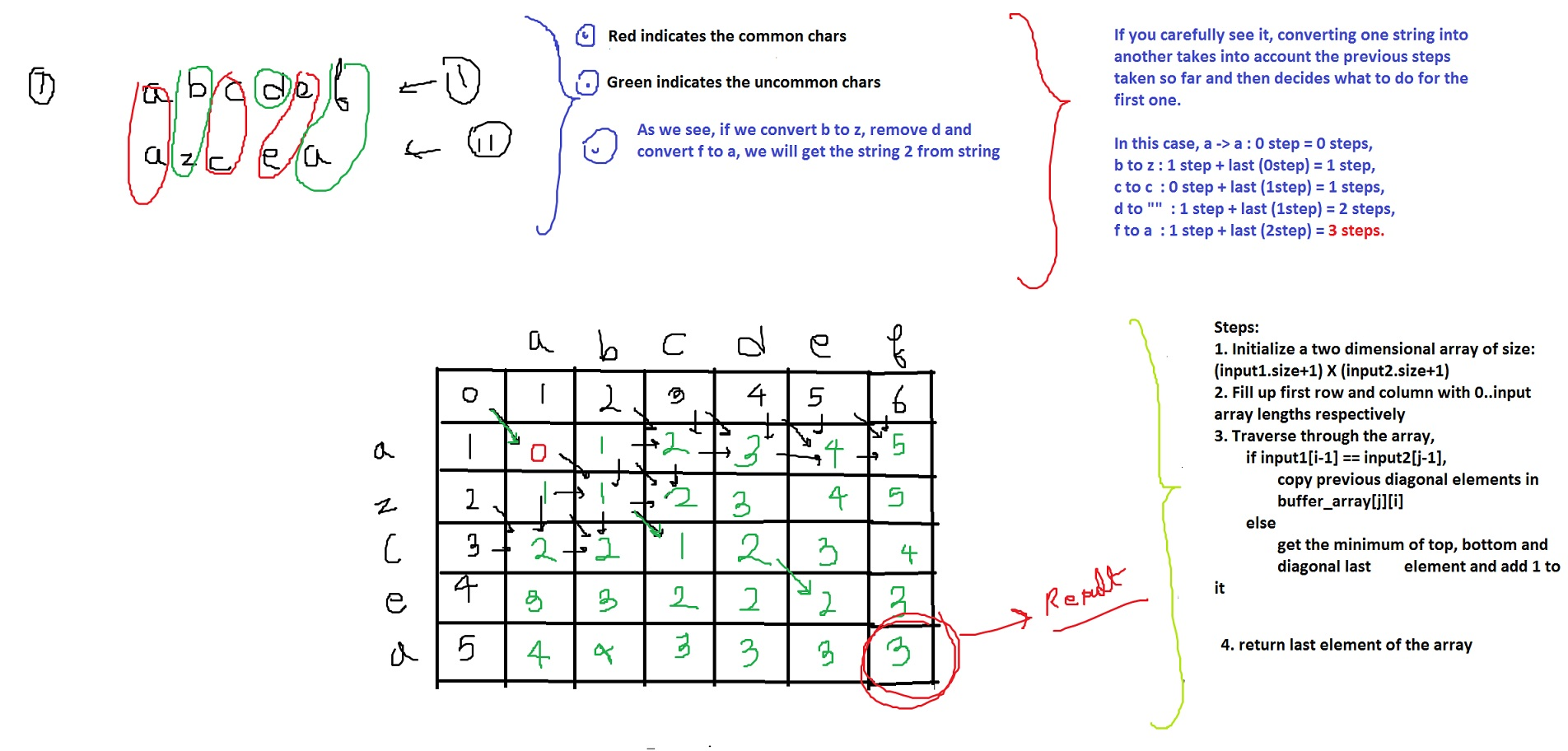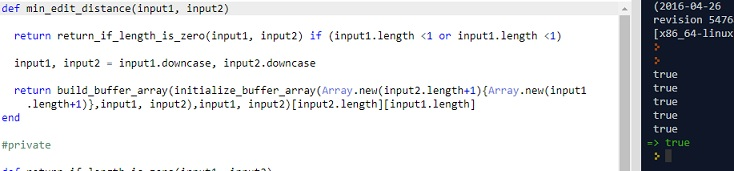# (*)CLEAN CODE: DYNAMIC PROGRAMMING: EDIT DISTANCE

By: Saurav

2017-11-13 22:37:00 UTC

Edit Distance
Given two words A and B, find the minimum number of steps required to convert A to B. (each operation is counted as 1 step.)

You have the following 3 operations permitted on a word:

Insert a character
Delete a character
Replace a character
Example :
edit distance between
"Anshuman" and "Antihuman" is 2.

Operation 1: Replace s with t.
Operation 2: Insert i.

InterviewBit

This is a very interesting problem and has direct implications in real world. e.g. DNA matching, Word Clustering algorithms.

The best way to solve it is using dynamic programming.

I have tried to visualize steps in the figure below:The image is self explanatory.

In a loop, compare elements of both input arrays.
If the input elements are equal, take the last diagonal element and add it to the 2d array,
because if the characters are equal, we don't need to take any steps
If the input elements are not equal, take the minimum of steps for the last character matching and add 1 to it.

If you want a better explanation, you should see Tushar Roy video as well. He explains it really well going through each steps slowly.
Lets go for the coding:

Lets write some tests first:

```p min_edit_distance('abcdef', 'azcea') == 3
p min_edit_distance("Anshuman","Antihuman") == 2
p min_edit_distance("","") == 0
p min_edit_distance("a","b") == 1
p min_edit_distance("asasaa","") == 6

```

### Attempt 1:

```def min_edit_distance(input1, input2)

if input1.length <1 and input1.length <1
return 0
elsif input1.length <1
return input2.length
elsif input1.length <1
return input1.length
end

input1, input2 = input1.downcase, input2.downcase

buffer_array = Array.new(input2.length+1){Array.new(input1.length+1)}

(0..input1.length).each do |i|
buffer_array[i] = i
end

(0..input2.length).each do |i|
buffer_array[i] = i
end

(1..input1.length).each do |i|
(1..input2.length).each do |j|
if input1[i-1] == input2[j-1]
buffer_array[j][i] = buffer_array[j-1][i-1]
else
buffer_array[j][i] = [buffer_array[j-1][i-1], buffer_array[j][i-1], buffer_array[j-1][i]].min+1
end
end
end

return buffer_array[input2.length][input1.length]

end
```

Looks ugly to me! Lets refactor it now.

### Attempt 2:

```def min_edit_distance(input1, input2)

return return_if_length_is_zero(input1, input2) if (input1.length <1 or input1.length <1)

input1, input2 = input1.downcase, input2.downcase

return build_buffer_array(initialize_buffer_array(Array.new(input2.length+1){Array.new(input1.length+1)},input1, input2),input1, input2)[input2.length][input1.length]
end

#private

def return_if_length_is_zero(input1, input2)
if input1.length <1 and input2.length <1
return 0
elsif input1.length <1
return input2.length
elsif input2.length <1
return input1.length
end
end

def initialize_buffer_array(buffer_array,input1, input2)
(0..input1.length).each do |i|
buffer_array[i] = i
end
(0..input2.length).each do |i|
buffer_array[i] = i
end
return buffer_array
end

def build_buffer_array(buffer_array,input1, input2)
(1..input1.length).each do |i|
(1..input2.length).each do |j|
input1[i-1] == input2[j-1] ? (buffer_array[j][i] = buffer_array[j-1][i-1]) : buffer_array[j][i] = [buffer_array[j-1][i-1], buffer_array[j][i-1], buffer_array[j-1][i]].min+1
end
end
return buffer_array
end
```All our tests pass. All our functions do one and only one thing.

And so we are done for now :)

### Let me know what you think! twitter: sprakash24octlinkedin

Owned & Maintained by Saurav Prakash

If you like what you see, you can help me cover server costs or buy me a cup of coffee though donation :)